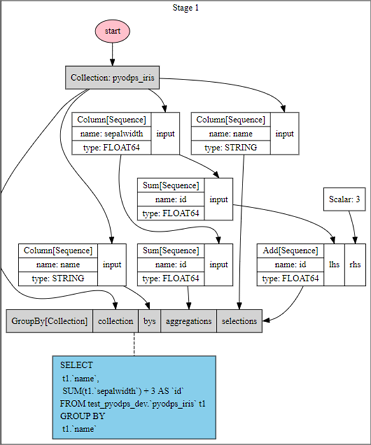Alibaba Cloud MaxCompute SDK for Python (PyODPS) DataFrame optimizes the execution process of each operation. You can use the visualization feature to display the entire computation process.

## DataFrame visualization

DataFrame visualization depends on graphviz and the graphviz package for Python.
``````>>> df = iris.groupby('name').agg(id=iris.sepalwidth.sum())
>>> df = df[df.name, df.id + 3]
>>> df.visualize()``````The computation process shows that PyODPS DataFrame combines the `groupby` operation and the column filtering operation.
``````>>> df = iris.groupby('name').agg(id=iris.sepalwidth.sum()).cache()
>>> df2 = df[df.name, df.id + 3]
>>> df2.visualize()``````The entire execution process runs in two steps because a cache operation is performed.

## View compiled results at the MaxCompute SQL backend

Call the `compile` method to view compiled results of SQL statements at the MaxCompute SQL backend.
``````>>> df = iris.groupby('name').agg(sepalwidth=iris.sepalwidth.max())
>>> df.compile()
Stage 1:

SQL compiled:

SELECT
t1.`name`,
MAX(t1.`sepalwidth`) AS `sepalwidth`
FROM test_pyodps_dev.`pyodps_iris` t1
GROUP BY
t1.`name```````

## Perform local debugging by using the pandas computation backend

If you use MaxCompute tables as the sources for DataFrame objects, the system does not compile or perform some operations at the MaxCompute SQL backend. Instead, the system uses the MaxCompute Tunnel service to download data. This way, the system does not need to wait for the MaxCompute SQL backend to schedule download tasks. This allows you to download small amounts of data from MaxCompute to a local directory and use the pandas computation backend to compile and debug code. You can perform the following debugging operations:
• Select all or some rows of data from a non-partitioned table, or filter the column data, and then calculate the number of specified rows. The column filtering operation does not compute column values.
• Select all or some rows of data from all or the first several partition columns that are specified in a partitioned table, or filter the column data, and then calculate the number of the specified rows.
Assume that the `iris` DataFrame object uses a non-partitioned MaxCompute table as the source. The following operation uses the MaxCompute Tunnel service to download data:
``````>>> iris.count()
>>> iris['name', 'sepalwidth'][:10]``````

Assume that the DataFrame object uses a partitioned table that includes the `ds`, `hh`, and `mm` partition fields. The following operation uses the MaxCompute Tunnel service to download data:

``````>>> df[:10]
>>> df[df.ds == '20160808']['f0', 'f1']
>>> df[(df.ds == '20160808') & (df.hh == 3)][:10]
>>> df[(df.ds == '20160808') & (df.hh == 3) & (df.mm == 15)]``````

In this case, you can use the `to_pandas` method to download the data to a local directory for debugging.

``````>>> DEBUG = True
>>> if DEBUG:
>>> df = iris[:100].to_pandas(wrap=True)
>>> else:
>>> df = iris``````

At the end of compiling, set `DEBUG` to False to complete computation on MaxCompute.

Note Some programs that pass local debugging may fail to run on MaxCompute because of the limits of the sandbox.